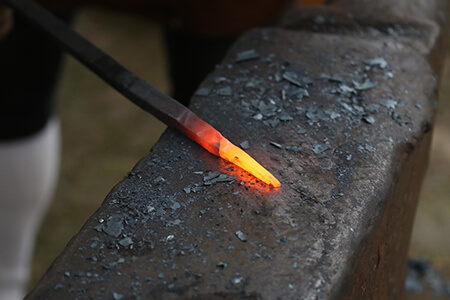Learning Objective

In this lesson we will learn how heat energy is transferred between substances by the processes of conduction, convection and radiation.

Learning Outcomes

By the end of this lesson you will be able to:

• Differentiate between heat energy and temperature.

• Define heat transfer.

• Explain how the processes of conduction, convection and radiation transfer heat energy, using examples.

• Describe thermal conductors and thermal insulators, and give examples of each.

• Explain how substances transmit, absorb and reflect infrared radiation differently.

## Contents

1 | Kinetic Energy, Heat Energy and Temperature

2 | Heat Transfer

3 | Conduction

4 | Convection

6 | Summary(Image: BlenderUnknown, Pixabay)

PREVIEW THE WORKSHEET FOR THIS LESSON

PREVIEW THE YEAR 9 PHYSICS WORKBOOK